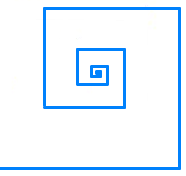# Run Cody Run!Cody has started running in a well-organized manner. He runs $100 \text{ m}$ east, then turns left and runs another $10 \text{ m}$ north, turns left and runs $1 \text{ m},$ again turns left and runs $0.1 \text{ m},$ and on the next turn $0.01 \text{ m},$ and so on.

Assuming that Cody can run in this pattern infinitely, the displacement from his initial position can be written as $\frac{a}{\sqrt{b}}$ with $a$ and $b$ being positive integers and $b$ square-free.

What is the value of $a \times b?$

×